#DAY 5
1
Software Development

## C# 如同精靈般優雅且高貴(下)

``````if (Math.Pow(16,2)*i+Math.Pow(16,1)*j+Math.Pow(16,0)*k == num)
{
var I =""; //先將要放入的字串宣告出來
if (i<10)
{
I = i.ToString(); //小於10的情況不用轉換,ToString()則是將數值轉換成字串的方法
}else{
switch (i)
{
case 10: I = "A"; break; //接著依照數值的不同會轉成不同的字串
case 11: I = "B"; break;
case 12: I = "C"; break;
case 13: I = "D"; break;
case 14: I = "E"; break;
case 15: I = "F"; break;
}
}
Console.WriteLine("{0} {1} {2}",I,j,k);
}
``````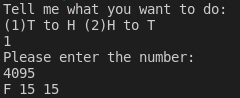### 工程師們就是一群懶惰蟲

``````static string returnAE(int number){
if (number<10)
{
return number.ToString();
}else{
switch (number)
{
case 10: return "A";
case 11: return "B";
case 12: return "C";
case 13: return "D";
case 14: return "E";
case 15: return "F";
default : return "";
}
}
}
``````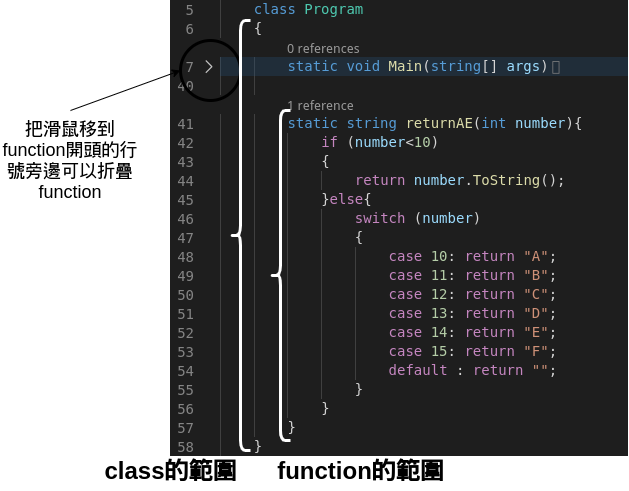• 方法在 C#裡面方法有分靜態跟非靜態(也有人稱動態)
靜態的話可以不用宣告就使用,詳細的分法我會在之後講物件的時候詳細說明,
現在就先記得 static 表示方法是靜態可以直接使用即可
• 回傳的型別表示當程式執行完後回傳值的型別,如果沒有回傳值就是 void 就可以了(之後會示範寫法),
這邊因為我們主程式(就是 main 那隻)需要知道代表各個數值的字串,因此需要有回傳值並且型別為字串(string)
• 方法名稱表示當你需要用這個方法的時候需要怎麼呼叫他
• 輸入值代表當你使用這個方法時需要輸入的數值(當然也可以不輸入,不過比較少見)
這邊因為我們需要將原本的數字轉成字串,因此輸入值的型別當然就是 int 拉,然後你可以重新命名你的變數

``````if (number<10)
{
return number.ToString();  //直接回傳,不用繼續執行後面的程式
}
``````

``````static string returnAE(int number){
if (number<10)
{
return number.ToString(); //小於10的在這裡就會回傳
}else{
switch (number)
{
case 10: return "A";
case 11: return "B";
case 12: return "C";
case 13: return "D";
case 14: return "E";
case 15: return "F"; //10～15會在這之前回傳
default : return ""; //其他的要在這裡回傳,就算是空值也一樣
}
}
}
``````

### 讓我們來使用剛熱好的方法吧

``````if (Math.Pow(16,2)*i+Math.Pow(16,1)*j+Math.Pow(16,0)*k == num)
{
Console.WriteLine("{0} {1} {2}",returnAE(i),returnAE(j),returnAE(k));
}
``````

``````returnAE(i)
``````

### 這樣我們的程式的功能就完成一半了

``````static void Main(string[] args)
{
Console.WriteLine("Tell me what you want to do:");
Console.WriteLine("(1)T to H (2)H to T");
switch (choose)
{
case "1":
t2h(number); //這裡拿出去做成方法
break;
case "2":
break;
default:
Console.WriteLine("Wrong selection");
break;
}
}

static void t2h(String number){
Int64 num = Int64.Parse(number);
for (int i = 0; i < 16; i++)
{
for (int j = 0; j < 16; j++)
{
for (int k = 0; k < 16; k++)
{
if (Math.Pow(16,2)*i+Math.Pow(16,1)*j+Math.Pow(16,0)*k == num)
{
Console.WriteLine("{0} {1} {2}",returnAE(i),returnAE(j),returnAE(k));
}
}
}
}
}
``````

``````switch (choose)
{
case "1":
t2h(number);
break;
case "2":
h2t(number);
break;
default:
Console.WriteLine("Wrong selection");
break;
}
``````

``````static void h2t(String input){
var pow = input.Length;
int i = 0;
while (i < pow)
{
Console.WriteLine(input[i]);
i++;
}
}
``````

``````var pow = input.Length;
``````

.Length 會把字串的長度取出來放入變數裡面,這邊我們的變數叫 pow

``````int i = 0;
while (i < pow)
{
Console.WriteLine(input[i]);
i++;
}
``````

while 是另外一種迴圈的結構,通常用於不是很明顯的知道實際的執行次數時

``````Console.WriteLine(input[i]);
``````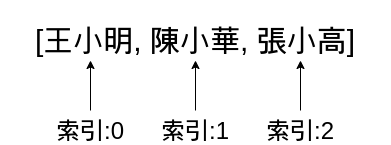OK, 讓我們來看看我們的輸入吧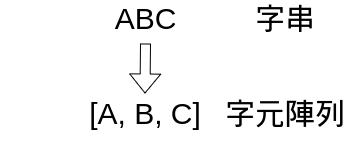就能找到 A(大部分的程式語言順序都是從 0 開始)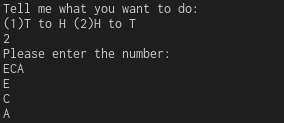``````static void h2t(String input){
double output = 0;
var pow = input.Length;
int i = 0;
while (i < pow)
{
output += AEreturn(input.Substring(i,1))*Math.Pow(16,pow-i-1);
i++
}
Console.WriteLine(output);
}

static int AEreturn(string number){
try {
return Convert.ToInt32(number);
}
catch{
switch (number){
case "A":
return 10;
case "B":
return 11;
case "C":
return 12;
case "D":
return 13;
case "E":
return 14;
case "F":
return 15;
default:
Console.WriteLine("Some thing wrong!");
return 0;
}
}
}
``````

``````static int AEreturn(string number){
``````

``````try{

}
catch{

}
``````

``````return Convert.ToInt32(number);
``````

``````switch (number){
case "A":
return 10;
case "B":
return 11;
case "C":
return 12;
case "D":
return 13;
case "E":
return 14;
case "F":
return 15;
default:
Console.WriteLine("Some thing wrong!");
return 0;
}
``````

### 好,回到方法 h2t 吧

``````static void h2t(String input){
double output = 0;
var pow = input.Length;
int i = 0;
while (i < pow)
{
output += AEreturn(input.Substring(i,1))*Math.Pow(16,pow-i-1);
i++
}
Console.WriteLine(output);
}
``````

double output = 0;

double 是一種型別,我們稱之為浮點數,為什麼要用他往下會提到

``````output += AEreturn(input.Substring(i,1))*Math.Pow(16,pow-i-1);
``````

+=? 這是什麼新符號?

``````i=i+2
``````

i 這個字必須重複打兩次

``````i+=2
``````

``````output = output + AEreturn(input.Substring(i,1))*Math.Pow(16,pow-i-1);
``````

``````AEreturn(input.Substring(i,1))  *  Math.Pow(16,pow-i-1);
``````

AEreturn 就是我們剛才完成的方法,裡面的

``````input.Substring(i,1)
``````

input[i]是直接去字串內取出某一個位置的字元,所以取出來的東西當然是字元

``````AEreturn(input.Substring(i,1))
``````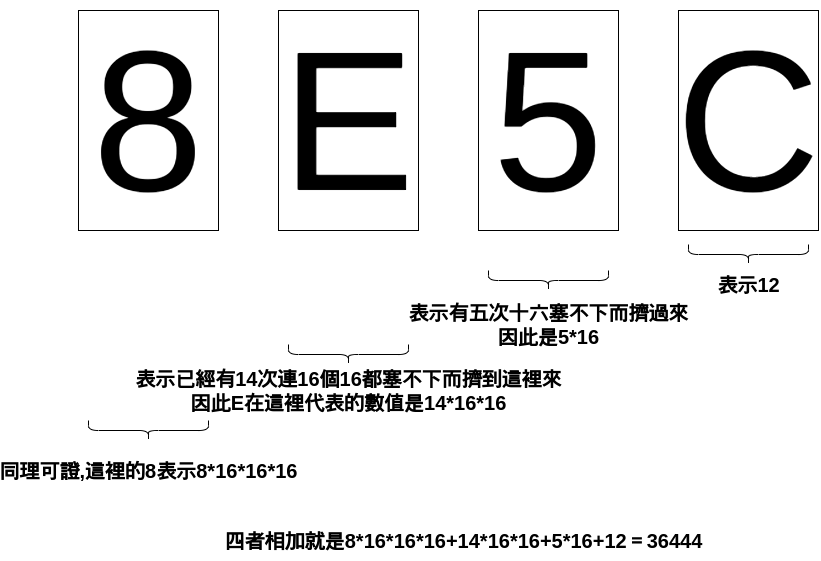``````Math.Pow(16,pow-i-1)
``````

### 在我們執行程式之前,讓我們玩一下字串跟字元的差異

``````while (i < pow)
{
Console.WriteLine(Convert.ToInt32(input[i])); //加上這一行
output += AEreturn(input.Substring(i,1))*Math.Pow(16,pow-i-1);
i++;
}
``````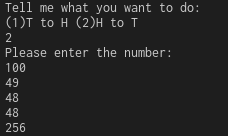``````dotnet run
``````

## 我們複習一下我們在 C#內學了什麼吧

• C#的程式結構
• 印出/讀取
• 邏輯控制
• switch,每一個條件使用break分開
• if ()裡面放條件,{}裡面放需要執行的程式
• 迴圈控制
• for 如果有明顯要執行幾次的時候用這個
• while 如果你當前不知道要執行幾次的時候可以用這個
• 創造自己的方法,回傳/不回傳值
• 字串轉型成 int,int 轉型成字串
• 計算上常用的冪次計算
• 異常處理

### 1 則留言

1

ultrahsin iT邦新手 5 級 ‧ 2021-05-16 21:36:37 檢舉

1. 部分h2t function中的while迴圈少了i++
2. "好，回到方法t2h吧"之標題推估是指h2t
skycover iT邦新手 5 級 ‧ 2021-05-17 17:24:37 檢舉